Miscellaneous

Chapter 5 Class 12 Continuity and Differentiability
Serial order wise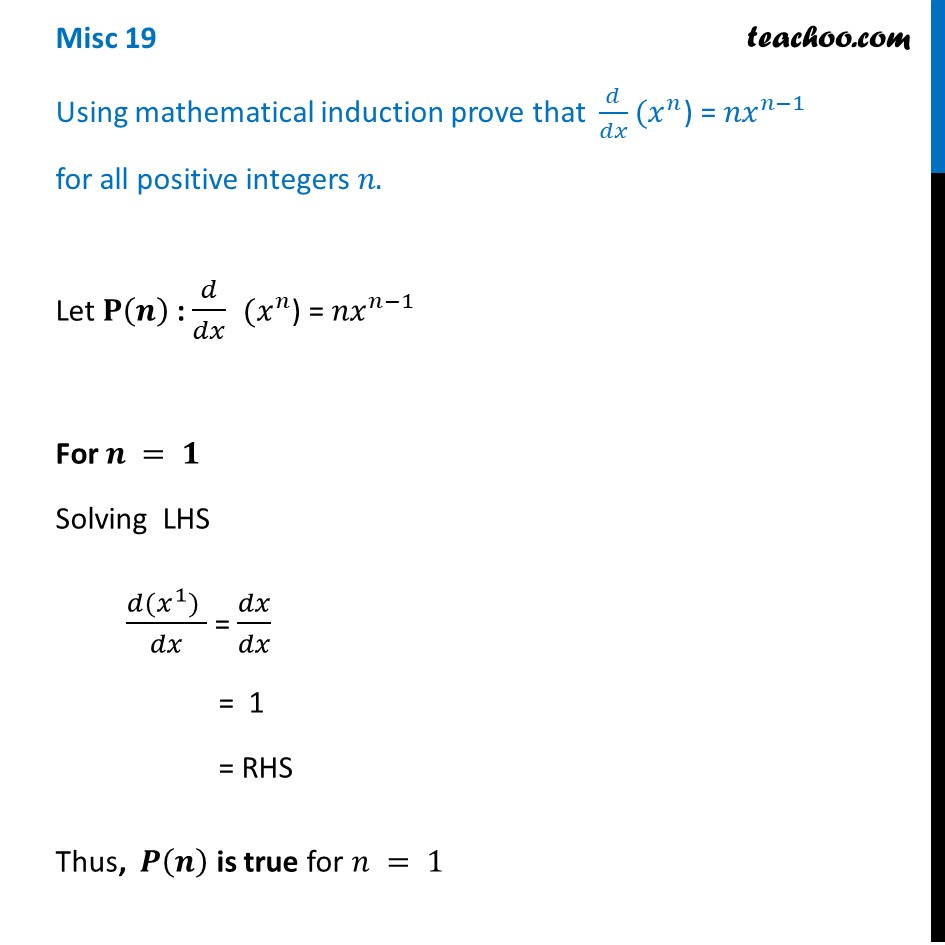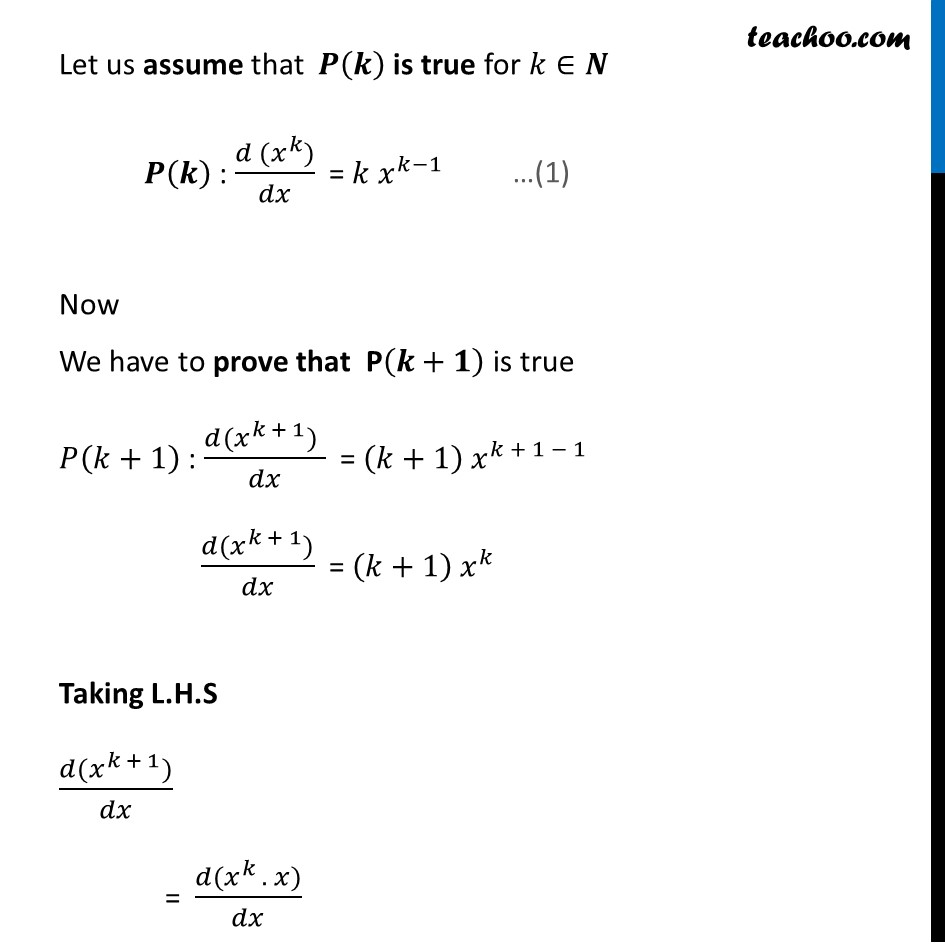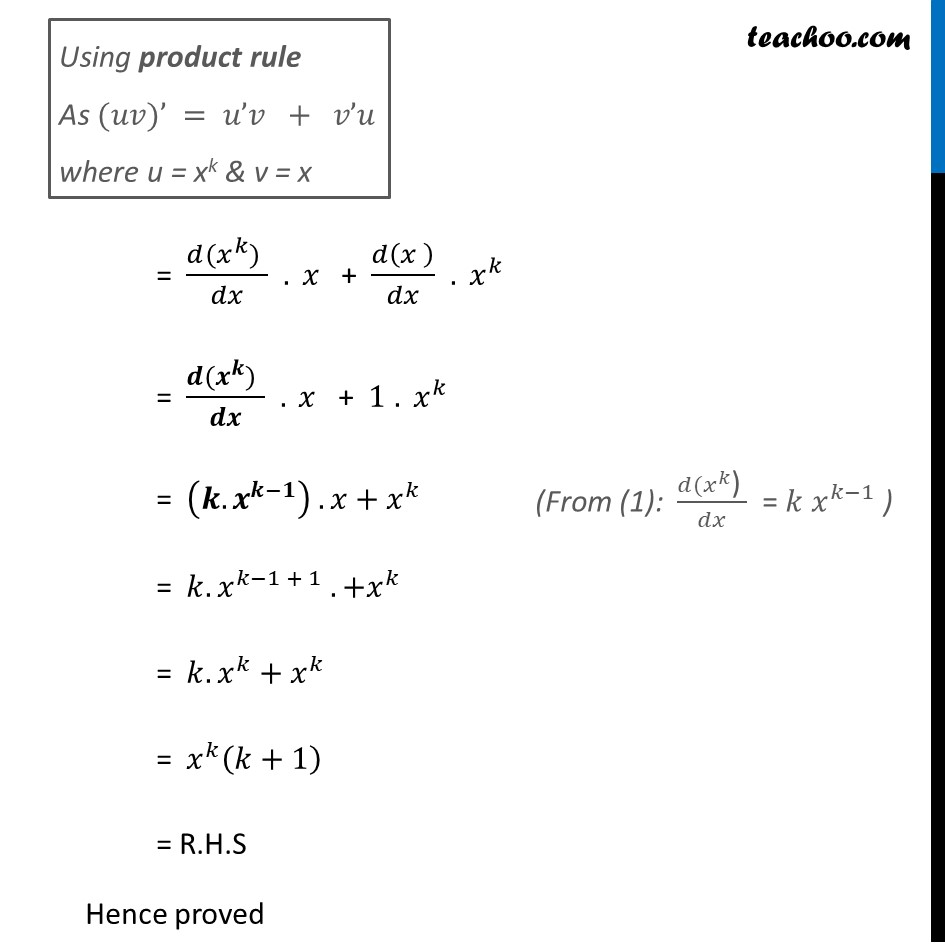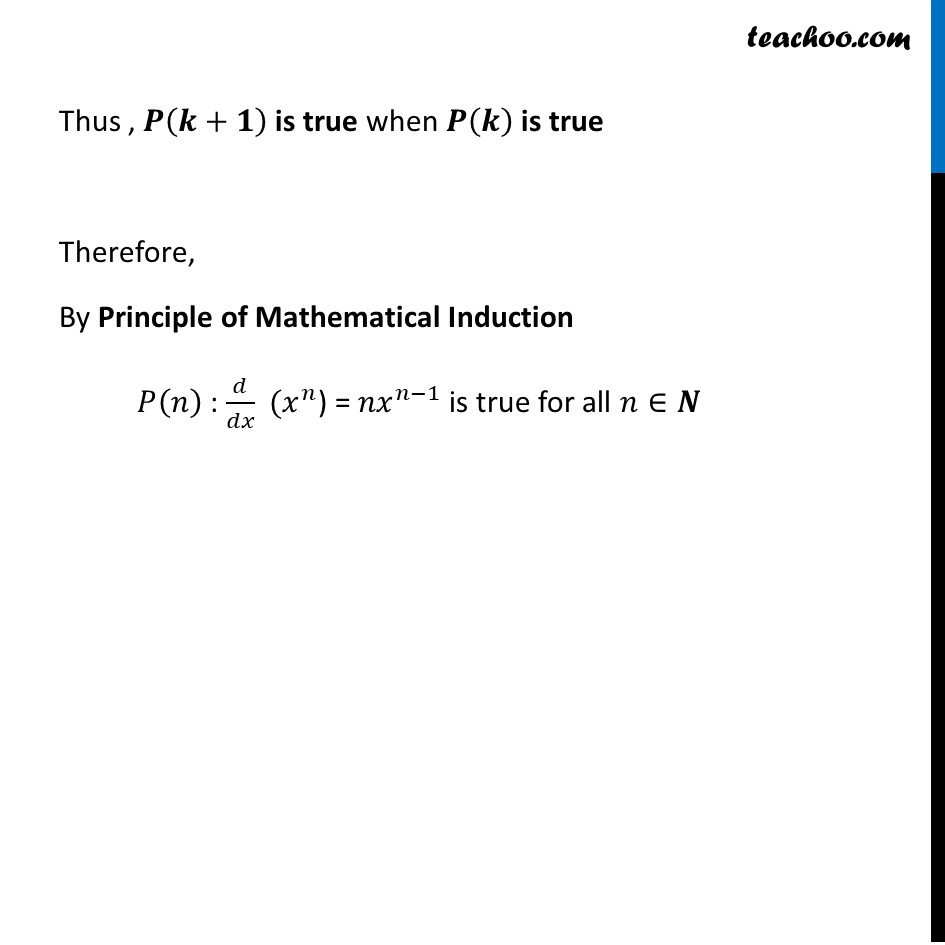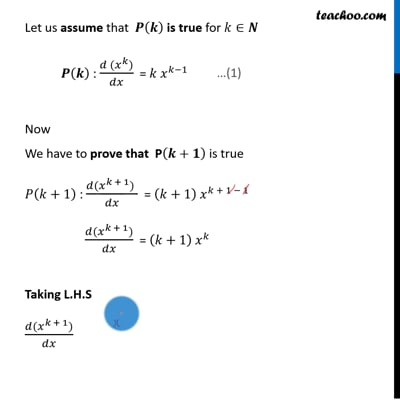This video is only available for Teachoo black users

Solve all your doubts with Teachoo Black (new monthly pack available now!)

### Transcript

Misc 19 Using mathematical induction prove that 𝑑/𝑑𝑥(𝑥^𝑛) = 〖𝑛𝑥〗^(𝑛−1) for all positive integers 𝑛. Let 𝐏(𝒏) : 𝑑/𝑑𝑥 (𝑥^𝑛) = 〖𝑛𝑥〗^(𝑛−1) For 𝒏 = 𝟏 Solving LHS (𝑑(𝑥^1)" " )/𝑑𝑥 = 𝑑𝑥/𝑑𝑥 = 1 = RHS Thus, 𝑷(𝒏) is true for 𝑛 = 1 Let us assume that 𝑷(𝒌) is true for 𝑘∈𝑵 𝑷(𝒌) : (𝑑 (𝑥^𝑘))/𝑑𝑥 = 〖𝑘 𝑥〗^(𝑘−1) Now We have to prove that P(𝒌+𝟏) is true 𝑃(𝑘+1) : (𝑑(𝑥^(𝑘 + 1))" " )/𝑑𝑥 = 〖(𝑘+1) 𝑥〗^(𝑘 + 1 − 1) (𝑑(𝑥^(𝑘 + 1)))/𝑑𝑥 = 〖(𝑘+1) 𝑥〗^𝑘 Taking L.H.S (𝑑(𝑥^(𝑘 + 1)))/𝑑𝑥 = (𝑑(𝑥^(𝑘 ). 𝑥))/𝑑𝑥 Using product rule As (𝑢𝑣)’ = 𝑢’𝑣 + 𝑣’𝑢 where u = xk & v = x = (𝑑(𝑥^𝑘)" " )/𝑑𝑥 . 𝑥 + 𝑑(𝑥 )/𝑑𝑥 . 𝑥^(𝑘 ) = (𝒅(𝒙^𝒌)" " )/𝒅𝒙 . 𝑥 + 1 . 𝑥^(𝑘 ) = (〖𝒌. 𝒙〗^(𝒌−𝟏) ) . 𝑥+𝑥^𝑘 = 〖𝑘. 𝑥〗^(𝑘−1 + 1) .+𝑥^𝑘 = 〖𝑘. 𝑥〗^𝑘+𝑥^𝑘 = 𝑥^𝑘 (𝑘+1) = R.H.S Hence proved (From (1): (𝑑(𝑥^𝑘 ") " )/𝑑𝑥 = 〖𝑘 𝑥〗^(𝑘−1) ) Thus , 𝑷(𝒌+𝟏) is true when 𝑷(𝒌) is true Therefore, By Principle of Mathematical Induction 𝑃(𝑛) : 𝑑/𝑑𝑥 (𝑥^𝑛) = 〖𝑛𝑥〗^(𝑛−1) is true for all 𝑛∈𝑵